Next: Example 8.3: Ampère's circuital Up: Magnetism Previous: Example 8.1: Earth's magnetic

## Example 8.2: Charged particle in magnetic field

Question: Suppose that an electron is accelerated from rest through a voltage difference of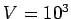volts and then passes into a region containing a uniform magnetic field of magnitude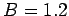T. The electron subsequently executes a closed circular orbit in the plane perpendicular to the field. What is the radius of this orbit? What is the angular frequency of gyration of the electron?

Answer: If an electron of mass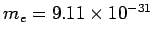kg and charge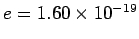C is accelerated from rest through a potential differencethen its final kinetic energy is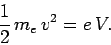Thus, the final velocityof the electron is given by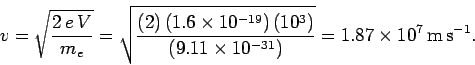The initial direction of motion of the electron is at right-angles to the direction of the magnetic field, otherwise the orbit of the electron would be a spiral instead of a closed circle. Thus, we can use Eq. (167) to calculate the radius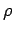of the orbit. We obtain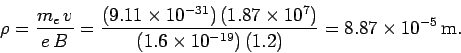The angular frequency of gyrationof the electron comes from Eq. (168):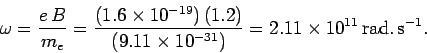Next: Example 8.3: Ampère's circuital Up: Magnetism Previous: Example 8.1: Earth's magnetic
Richard Fitzpatrick 2007-07-14